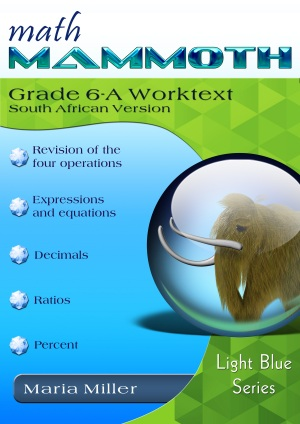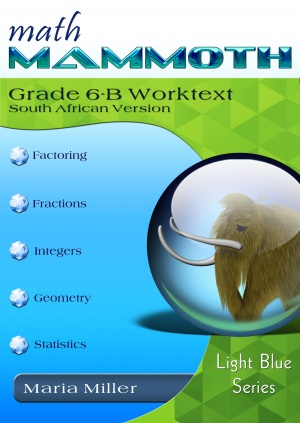^

You are here: HomeMM South African Version → Grade 6

# Math Mammoth Grade 6, South African version

Math Mammoth Grade 6, South African Version is a complete maths program for 6th grade. It consists of two student worktexts (A and B), a separate answer key for each worktext, tests, mixed revisions, and an additional worksheet maker (Internet access required) to make extra practice worksheets when needed.179 pages
160 lesson pages175 pages
150 lesson pages

## Prices & ordering

Grade 6 full set: USD \$37.50

Includes part A and B worktexts, answer keys, tests, mixed revisions, a worksheet maker, and Soft-Pak.

You will be purchasing the download from my authorized reseller Comecero, LLC.

At the heart of the curriculum are two student worktexts (A and B), each covering about half a year of maths work. These worktexts contain all the instruction and the exercises in the same book — which makes lesson preparation a breeze. The worktexts are written directly to the student, and thus they allow many students to teach themselves and to learn directly from the books.

You will also get separate answer keys, chapter tests, additional cumulative reviews (I do recommend you use at least some of them), and a versatile worksheet maker (Internet access required) for those times when your child needs just a bit more practice.

## Features

• Math Mammoth focuses on conceptual understanding. It explains the "WHY", so your children can understand the maths, not just learn "HOW" to do it.
• The curriculum is mastery-oriented. This means it concentrates fairly long on a topic, delving into its various aspects. This promotes conceptual understanding, as opposed to spiral curricula that often tend to jump from topic to topic too much.
• The curriculum has no separate teacher's manual, nor is it scripted. The introduction to each chapter has some notes for the teacher concerning the material in the chapter. All the instruction is written directly to the student in the worktext, and there also exist accompanying videos where you can see Maria herself teach the material.
• Very little teacher preparation is required.• Concepts are often explained with visual models, followed by exercises using those models. These visual models can take the place of manipulatives for many children; however, it is very easy to add corresponding manipulatives to the lessons if so desired.
• After each chapter introduction, you will find a list of Internet links and resources (games, quizzes, animations, etc.) that can be used for fun, illustrations, and further practice.

• The PDF files can be filled in on a computer, phone, or tablet, using the annotation tools found in many PDF apps. Learn more.
• BONUS! The digital download also comes with BONUS Soft-Pak programs. The 6 programs in Soft-Pak (4 math, 1 language arts, 1 list maker) offer both on-screen and printable activities in a low-graphic, high content format. Read more and see screenshots.

## Overview of topics

The main areas of study in Math Mammoth Grade 6 are:

• review of the basic operations with whole numbers
• beginning algebra topics: expressions, equations and inequalities
• review of all decimal arithmetic
• introduction to ratios and percent
• prime factorization, GCF and LCM
• a review of fraction arithmetic from 5th grade, plus a focus on division of fractions
• the concept of integers, coordinate grid, addition & subtraction of integers
• geometry: review of quadrilaterals & drawing problems; area of triangles & polygons; volume of rectangular prisms with fractional edge lengths; surface area
• statistics: concept of distribution, measures of center, measures of variation, summarizing distributions, boxplots, stem-and-leaf plots, histograms

Please also see the tables of contents in the sample files, which will let you see the topics covered in more detail.

## South Africa

Math Mammoth South African Version has been customised to South Africa in the following manners:

• The names used are South African names (such as Ansie and Jali).
• The currency used in word problems is rand. The money chapter teaches both rand and cents.
• The material is "all metric". In other words, the US customary measuring units are not used.
• Spelling is British English instead of American English.
• Paper size is A4.
• Geographic locations used emphasise South African locations (such as Pretoria, Johannesburg).

Please note that the curriculum is not following the South African official syllabus for 4th grade maths. Instead, it simply is a copy of the US version of Math Mammoth Grade 4. For the most part, Math Mammoth exceeds South African standards. Some standards may not be covered.

## Skills Review Workbook

Math Mammoth Skills Review Workbook, Grade 6 provides additional practice for the topics in Math Mammoth Grade 6 curriculum. I recommend you only purchase it once you are sure your student does need supplemental practice. Learn more.

## Introduction

In sixth grade, students encounter the beginnings of algebra, learning about algebraic expressions, one-variable equations and inequalities, integers, and ratios. We also review and deepen the students' understanding of rational numbers: both fractions and decimals are studied in depth, while percent is a new topic for 6th grade. In geometry, students learn to compute the area of various polygons, and also calculate volume and surface area of various solids. The last major area of study is statistics, where students learn to summarize and describe distributions using both measures of center and variability.

The year starts out with a review of the four operations with whole numbers (including long division), place value, and rounding. Students are also introduced to exponents and do some problem solving.

Chapter 2 starts the study of algebra topics, delving first into expressions and equations. Students practice writing expressions in many different ways, and use properties of operations and the idea of maintaining the equality of both sides of an equation to solve simple one-step equations. We also study briefly inequalities and using two variables.

Chapter 3 has to do with decimals. This is a long chapter, as we review all of decimal arithmetic, just using more decimal digits than in 5th grade. Students also convert measuring units in this chapter.

Ratios (chapter 4) is a new topic for sixth grade. Students are already familiar with finding fractional parts from earlier grades, and now it is time to advance that knowledge into the study of ratios, which arise naturally from dividing a quantity into many equal parts. We study such topics as rates, unit rates, equivalent ratios, and problem solving using bar models.

Percent (chapter 5) is an important topic to understand thoroughly, because of its many applications in real life. The goal of this chapter is to develop a basic understanding of percent, to see percentages as decimals, and to learn to calculate discounts.

In chapter 6, we first review prime factorization, and then study the greatest common factor and the least common multiple.

Chapter 7 first presents a thorough review of fraction operations from 5th grade (addition, subtraction, and multiplication). The topic of focus in the chapter is fraction division. Students also do some problem solving with fractions.

In chapter 8, students are introduced to integers. They plot points in all four quadrants of the coordinate plane and learn to add and subtract with negative numbers. Multiplication and division of integers are studied in 7th grade.

In geometry (chapter 9), the focus is on the area of polygons. This is studied in a logical progression: first, the area of right triangles, then the area of parallelograms, then, the area of triangles, and lastly the area of polygons. We also review some topics from earlier grades (quadrilaterals, triangles, perimeter, basic drawing). Other major topics in this chapter are calculating surface area of some solids using nets, and volume of rectangular prisms with fractional edge lengths.

Lastly, in chapter 10, students study statistics. The focus idea is that of a statistical distribution. Students learn about measures of center and measures of variability. They learn how to make dot plots, histograms, boxplots, and stem-and-leaf plots, and how to summarize distributions.

## Manipulatives

Here is a list of manipulatives, or actually just measurement tools, that are needed for grade 6.

1. A ruler that measures in centimetres
2. A protractor.

## Reviews / Testimonials of the 6th grade curriculum

Taking a look at Math Mammoth — a review of MM6 by Jaime Gravitt at My Homeschool Story blog

Dear Maria,
You are awesome. Thanks so much for your amazing curriculum. I am using 6th and 7th grade books for my 7th grade daughter who is homeschooling this year. I love that your curriculum corresponds to state standards so when my daughter goes back to school she not be behind. My daughter has gone from a student who did not like math to one who does math first thing in the morning! Thanks again!!

WAIT!

Receive my monthly collection of math tips & resources directly in your inbox — and get a FREE Math Mammoth book!We respect your email privacy.
You can unsubscribe at any time.

### Math Mammoth Tour

Confused about the different options? Take a virtual email tour around Math Mammoth! You'll receive:

An initial email to download your GIFT of over 400 free worksheets and sample pages from my books. Six other "TOURSTOP" emails that explain the important things and commonly asked questions concerning Math Mammoth curriculum. (Find out the differences between all these different-colored series!)

This way, you'll have time to digest the information over one or two weeks, plus an opportunity to ask me personally about the curriculum.
A monthly collection of math teaching tips & Math Mammoth updates (unsubscribe any time)We respect your email privacy.

Note: You will FIRST get an email that asks you to confirm your email address. If you cannot find this confirmation email, please check your SPAM/JUNK folder.

### "Mini" Math Teaching Course

This is a little "virtual" 2-week course, where you will receive emails on important topics on teaching math, including:

- How to help a student who is behind
- Troubles with word problems
- Teaching multiplication tables
- Why fractions are so difficult
- The value of mistakes
- Should you use timed tests
- And more!

You will also receive:

A GIFT of over 400 free worksheets and sample pages from my books right in the very beginning.A monthly collection of math teaching tips & Math Mammoth updates (unsubscribe any time)
We respect your email privacy.

Note: You will FIRST get an email that asks you to confirm your email address. If you cannot find this confirmation email, please check your SPAM/JUNK folder.

### Maria's Math Tips

Enter your email to receive math teaching tips, resources, Math Mammoth news & sales, humor, and more! I tend to send out these tips about once monthly, near the beginning of the month, but occasionally you may hear from me twice per month (and sometimes less often).You will also receive:

• A GIFT of over 400 free worksheets and sample pages from my books.We respect your email privacy.